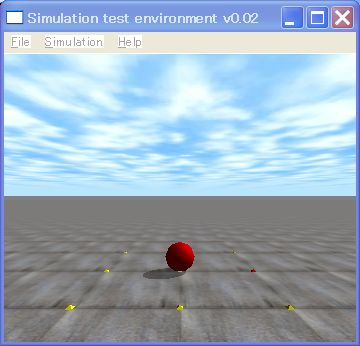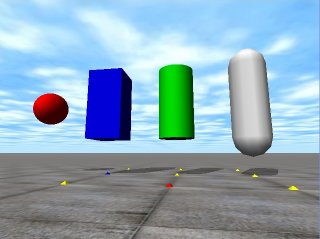# 6. Collision DetectionThis is the 6th ODE (Open Dynamics Engine) tutorial.

This time, I explain the collision detection. In the previous tutorial, as you know, dynamics and the collision detection are separately implemented in ODE.To calculate the dynamics, firstly create a world by dWorldCreate (), secondly create a body  in the world, and finally calculate the dynamics by  dWorldStep().

Geometry

A geometry is a shape of an object.  It is used for collision detection. The below figure shows typical geometries, such as  sphere, box, cylinder and capsule.Meanwhile, in order to calculate the collision detection,firstly create a space by dHashSpaceCreate (), secondly create a geometry  in the space, and finally detect a collision by dSpaceCollide().

In the following sample program, In line 97,  a sphere geometry is created by dCreateSphere()In line 98, ball.geom, the geometry of the ball, is associated with ball.body by dGeomSetBody(). An ODE object is composed of body and geometry. Both attributes must be associated.

Then, dSpaceCollide()is called in the simLoop function.  Arguments o1 and o2 of nearCallback() are geometries which  are potentially collide each other.  You can set properties, such as the upper number of contact points, a friction coefficient,  a bounce coefficient, a friction model, a softness parameter, and so on,  of the contact surface in the nearCallback() function.

In addition, you have to be careful that the arguments  o1 and o2 probably  collide, sometimes these do not collide each other. In order to know the collision, examine return value from dCollide(). If there is the collision, the return value is more than one.

As in line 88, firstly create a contact group by dJointGroupCreate() which is a container of collision points,  and clear the container by dJointGroupEmpty() every simulation step as in line 53.

Now, let’s read the source code.

```// sample6.cpp
#include <ode/ode.h>
#include <drawstuff/drawstuff.h>

static dWorldID world;
static dSpaceID space;
static dGeomID  ground;
static dJointGroupID contactgroup;
static int flag = 0;
dsFunctions fn;

const dReal   mass   = 1.0;

typedef struct {
dBodyID body;
dGeomID geom;
} MyObject;
MyObject ball;

static void nearCallback(void *data, dGeomID o1, dGeomID o2)
{
const int N = 10;
dContact contact[N];

int isGround = ((ground == o1) || (ground == o2));

int n =  dCollide(o1,o2,N,&contact.geom,sizeof(dContact));

if (isGround)  {
if (n >= 1) flag = 1;
else        flag = 0;
for (int i = 0; i < n; i++) {
contact[i].surface.mode = dContactBounce;
contact[i].surface.mu   = dInfinity;
contact[i].surface.bounce     = 0.0; // (0.0~1.0) restitution parameter
contact[i].surface.bounce_vel = 0.0; // minimum incoming velocity for bounce
dJointID c = dJointCreateContact(world,contactgroup,&contact[i]);
dJointAttach (c,dGeomGetBody(contact[i].geom.g1),dGeomGetBody(contact[i].geom.g2));
}
}
}

static void simLoop (int pause)
{
const dReal *pos,*R;

flag = 0;
dSpaceCollide(space,0,&nearCallback);

dWorldStep(world,0.01);

dJointGroupEmpty(contactgroup);

if (flag == 0) dsSetColor(1.0, 0.0, 0.0);
else           dsSetColor(0.0, 0.0, 1.0);
pos = dBodyGetPosition(ball.body);
R   = dBodyGetRotation(ball.body);
}

void start()
{
static float xyz = {0.0,-3.0,1.0};
static float hpr = {90.0,0.0,0.0};
dsSetViewpoint (xyz,hpr);
}

void  prepDrawStuff() {
fn.version = DS_VERSION;
fn.start   = &start;
fn.step    = &simLoop;
fn.command = NULL;
fn.stop    = NULL;
fn.path_to_textures = "../../drawstuff/textures";
}

int main (int argc, char *argv[])
{
dReal x0 = 0.0, y0 = 0.0, z0 = 2.0;
dMass m1;

prepDrawStuff();

dInitODE();
world = dWorldCreate();
space = dHashSpaceCreate(0);
contactgroup = dJointGroupCreate(0);

dWorldSetGravity(world,0,0,-0.5);

// Create a ground
ground = dCreatePlane(space,0,0,1,0);

// Create a ball
ball.body = dBodyCreate(world);
dMassSetZero(&m1);
dBodySetMass(ball.body,&m1);
dBodySetPosition(ball.body, x0, y0, z0);

dGeomSetBody(ball.geom,ball.body);

dsSimulationLoop (argc,argv,352,288,&fn);

dWorldDestroy (world);
dCloseODE();

return 0;
}```

In the code, a body and a geomerty are member of the MyObject structure.

dSpaceCollide(), the collision detection function, is called in the simLoop function. You must call it before dWorldStep(). dSpaceCollide() finds contact points and they are constraint for the dynamics calculaiton.

dSpaceCollide() calls the callback function nearCallback().  dSpaceCollide() passes two geometries, o1 and o2,  which potentially collide to nearCallback(). The return value of dCollide() is the number of contact points in line 28.

In line 30,  if (isGround) sentence from line 30 to line 41, only geometries which potentially collide are processed.In other words, we do not care geometries which are not ground.  Please uncomment the if sentence, if you want to detect collisions of geometries which are not ground.

See you next time!

• APIs for collision detection
• dSpaceID dHashSpaceCreate(0)Create a space. Return value is the space ID.
• dGeomID dCreatePlane (dSpaceID space, dReal a, dReal b, dReal c, dReal d)Create a plane. Arguments are parameters of the plane equation  ax+by+cz = d.
• dGeomID dCreateSphere (dSpaceID space, dReal r)Create a sphere, r is a radius of the sphere.
• void dGeomSetBody (dGeomID geom, dBodyID body)This API associates a geometry with a body.
• dJointGroupID dJointGroupCreate (0)Create a joint group which is a container of contact points.
• void dJointGroupEmpty (dJointGroupID)Empty a  joint group.  This API must be called in every simulation step.
スポンサーリンク
demura.netをフォローするdemura.net

### Folding@home Kanazawa (ID 257261)

みんなのためにおうちで新型コロナウイルスを解析しよう！

タイトルとURLをコピーしました